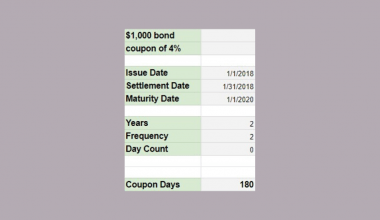# How to Use the TO_PERCENT Function in Google Sheets

The TO_PERCENT function in Google Sheets is used to convert a provided number into a percentage.

This function is useful because it helps you understand a certain piece of information in terms of its percentage, without having to fumble around with extra calculations in your formula and formatting the result to the correct percent number format right off the bat. It provides an easy way to calculate the percentage with the given information.

Let’s look at an example.

You have information about some of the debts you are slowly paying off over the course of a few months. You have the information about how much the value of each debt is, and how much you have paid for each debt to date. What are the percentage completion rates for each?

Many people will instinctively divide the payment over the entire debt, and multiply the result by 100, and then adjust the formatting of the cell to make it a percentage figure.

The `TO_PERCENT` function takes in all these inputs in order to calculate the percentage rate, without having to create complex formulas where you need to explain the logic in some parts and avoid making small mistakes that can affect your entire spreadsheet.

## The Anatomy of the TO_PERCENT Function in Google Sheets

The syntax of the `TO_PERCENT` function is as follows::

`=TO_PERCENT(value)`

Let’s have a look at each part of the function to understand what is going on here:

• `=` is the equals sign that starts off any function in Google Sheets.
• `TO_PERCENT` is the name of our function.
• `value` is the argument or reference to a cell to be converted to a percentage.

Note that if the given `value` is a number or reference to a cell containing a numeric value, it outputs the percentage with the standard interpretation that 1 = 100%. If the `value` isn’t a number, it returns the number without modification.

This formula also works on number formats like dates and other percentage figures, so be aware of the format of the cells you are performing on.

## A Real Example of Using the TO_PERCENT Function

Let’s look at the example below to see how to use `TO_PERCENT` function in Google Sheets.

#### Converting to Percentage Rate in Google Sheets

This is a simple problem. We want to change any applicable number to a percentage.

``

The function takes just one argument. So in the equation, it will look like:

`=TO_PERCENT(F3)`

In this problem, we basically need to convert the long decimal in cell E3 into a percentage. The instinct of many people would be to multiply the ratio by 100, and then click on Format > Number > Percent.

The good thing is `TO_PERCENT` removes these two extra steps from the equation, saving you some time calculating the figure and making future adjustments in your spreadsheet.

As a result, we get 39%.

This simple problem can be practiced to perfection. Use the link below to get a copy of this problem set:

## How to Use the TO_PERCENT Function in Google Sheets

In this section, we will show you a step-by-step process on how to use the `TO_PERCENT` function in Google Sheets.

In this problem, we will be calculating the percentage rate.

#### Calculating the Percentage Rate in Google Sheets

1. To begin, click on a cell to make active, which you would like to display `TO_PERCENT`. For this guide, The `TO_PERCENT` rate will be in Cell E3.1. Next, type the equal sign ‘=’ to start writing the function. Follow this with “TO_PERCENT” or “to_percent” – Google Sheets functions are not case sensitive, so either is fine.1. The auto-suggest box will create a drop-down menu. Select the TO_PERCENT function by clicking it. It is the first to pop up on the list, but take care to choose the correct function.1. After the opening bracket ‘(‘, you will add the value attribute. First, we input the actual number of words written. You can input the value or cell reference. Cell reference makes the sheet more dynamic and neat, so that’s what we will go for in this example.1. Next, we input the goal number of words written. You can input the value or cell reference. Cell reference makes the sheet more dynamic and neat, so that’s what we will go for in this example. You can even see a preview of the calculation.1. Hit Enter, and the result should appear.1. Since we created a dynamic formula, just drag the formula down to the other cells.1. That’s it – you are now done!Given a practical problem where you should solve and present the correct percentage rate, use the `TO_PERCENT` function can help tidy up and automate your spreadsheet. This should definitely help you avoid mistakes in your spreadsheets in the future, too.

And there you have it – you can now use the `TO_PERCENT` function in Google Sheets together with the other numerous Google Sheets formulas to create even more effective formulas.Our goal this year is to create lots of rich, bite-sized tutorials for Google Sheets users like you. If you liked this one, you'll love what we are working on! Readers receive ✨ early access ✨ to new content. There will be no spam and you can unsubscribe at any time.

##### You May Also Like## How to Use LEFT Function in Google Sheets

The LEFT function in Google Sheets is useful to return a substring from the beginning of a specified…## How to Compare Two Sheets in Google Sheets

Learning how to compare two sheets in Google Sheets is useful to identify matches and differences between two…## How to Use DATEDIF Function in Google Sheets

The DATEDIF function in Google Sheets is useful if you want to know how far two different given…## How to Use COUPDAYS Function in Google Sheets

Knowing how to use the COUPDAYS function in Google Sheets is useful if you want to calculate the…